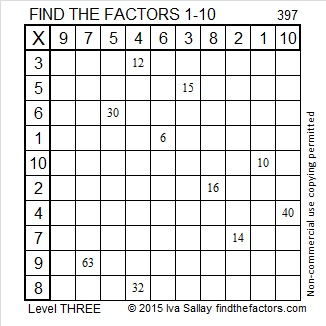# 397 and Level 3

Let’s do a quick test to see if 397 is a prime number or a composite number: 2^397 (mod 397) = 2, so 397 is VERY LIKELY a prime number. Scroll down past the puzzle to know for sure.Print the puzzles or type the factors on this excel file: 10 Factors 2015-02-16

• 397 is a prime number.
• Prime factorization: 397 is prime.
• The exponent of prime number 397 is 1. Adding 1 to that exponent we get (1 + 1) = 2. Therefore 397 has exactly 2 factors.
• Factors of 397: 1, 397
• Factor pairs: 397 = 1 x 397
• 397 has no square factors that allow its square root to be simplified. √397 ≈ 19.9248588How do we know that 397 is a prime number? If 397 were not a prime number, then it would be divisible by at least one prime number less than or equal to √397 ≈ 19.925. Since 397 cannot be divided evenly by 2, 3, 5, 7, 11, 13, 17, or 19, we know that 397 is a prime number.

19² + 6² = 397 and 397 is the hypotenuse of primitive Pythagorean triple 228-325-397.

A Logical Approach to FIND THE FACTORS: Find the column or row with two clues and find their common factor. Write the corresponding factors in the factor column (1st column) and factor row (top row).  Because this is a level three puzzle, you have now written a factor at the top of the factor column. Continue to work from the top of the factor column to the bottom, finding factors and filling in the factor column and the factor row one cell at a time as you go.